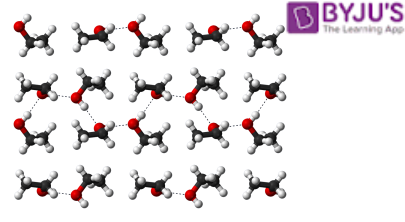# Determination of Molecular Weight using Colligative Properties

The use of colligative properties of solution in the determination of the molecular weight of various compounds has been one of the simplest methods. Since the colligative properties of solutions depend only on the number of molecules of solute in them, this method is especially used for determining the molar masses of complex molecules, proteins, macromolecules, and polymers.
A solution of known concentration of the substance is prepared in a solvent whose boiling point or freezing point or vapour pressure is known to us. The property to be measured is chosen in such a way that its measurement is simple and easy under the given conditions and does not vary considerably.Let us consider the colligative properties one by one and molecular weight calculation based on these properties.

## 1. Elevation of Boiling Point

We know that the boiling point of a pure solvent rises when a non-volatile solute is added to it. This elevation in boiling point is proportional to the molal concentration of the solute in the solution. Mathematically it is given as:
$$\begin{array}{l} ∆T_b=K_b m \end{array}$$

Where,

$$\begin{array}{l} ΔT_b = elevation\ in\ boiling\ point\end{array}$$
$$\begin{array}{l}K_b = Boiling\ Point\ Elevation\ Constant\end{array}$$

m = molal concentration of the solution we know that molality is the number of moles of solute per

Now we know that molality is the number of moles of solute per kilogram of the solvent. Let the weight of solute be w2 having molar mass M2 dissolved in w1 grams of solvent. Then molality can be given as:

Then molality can be given as:

$$\begin{array}{l}m= \frac {moles ~of~ solute}{mass ~of~ solvent~ in ~Kg} = \frac {w_2/M_2}{w_1/1000} \end{array}$$

$$\begin{array}{l}m= \frac {moles ~of~ solute}{mass ~of~ solvent~ in ~Kg} = \frac {w_2/M_2}{w_1/1000} \end{array}$$

$$\begin{array}{l} \Rightarrow m = \frac {1000 × w_2}{w_1 × M_2} \end{array}$$

So now, elevation of boiling point is given by:

So now, the elevation of boiling point is given by:

$$\begin{array}{l} ∆T_b = \frac {K_b × 1000 × w_2}{w_1 × M_2} \end{array}$$

Now if we rearrange the above equation, we get:

$$\begin{array}{l} \Rightarrow M_2 = \frac {K_b~ × ~1000~× ~w_2}{w_1 ~×~ \triangle T_b} \end{array}$$

The above equation gives us a relation between molecular weight and the colligative property which is the elevation in boiling point.

## 2.   Depression of Freezing Point

The depression at the freezing point is another colligative property of solutions that gives us a method to determine the molar masses of various substances similar to the elevation of the boiling point. We know that depression at the freezing point is given as:

$$\begin{array}{l} ∆T_b=K_f m \end{array}$$

Where,

$$\begin{array}{l} ΔT_f = depression\ of\ freezing\ point\end{array}$$
$$\begin{array}{l}K_f = Freezing\ Point\ Constant\end{array}$$
m = molal concentration of the solution

From the above description we now know that molality is given as:

$$\begin{array}{l} m = \frac {1000 × w_2}{w_1 × M_2} \end{array}$$

Therefore,
Freezing point depression can be written as:

$$\begin{array}{l} ∆T_f = \frac { K_f × 1000 × w_2}{w_1 × M_2 } \end{array}$$

Now if we rearrange the above equation, we get:

$$\begin{array}{l} => M_2 = \frac {K_f × 1000 × w_2}{w_1 × ∆T_f} \end{array}$$

Thus we can calculate the molar mass of a solute as per the above equation if we can measure the depression in the freezing point of the solvent on the addition of the solute.

## 3. Osmotic Pressure

Osmotic Pressure is the pressure applied to just stop the flow of solvent molecules through a semi-permeable membrane from a dilute solution to a concentrated solution.

Mathematically it is given as:

π=CRT

Where,
π =osmotic pressure
C= Molar concentration of the solution
R= Universal gas constant
T= Temperature
Now molar concentration of a solution containing w2 grams of solute whose molar mass is
$$\begin{array}{l} M_2 \end{array}$$
, and the total volume of the solution is V litres, can be written as:

$$\begin{array}{l} C = \frac {w_2/M_2}{V} = \frac {w_2}{V × M_2 } \end{array}$$

So we can now write the osmotic pressure as:

So we can now write the osmotic pressure as:

$$\begin{array}{l} π = \frac {w_2 RT}{M_2 V} \end{array}$$

Rearranging the above equation we get,

$$\begin{array}{l} M_2 = \frac {w_2 RT}{πV} \end{array}$$

Thus we can calculate the molecular weight of a substance using the colligative properties of solutions. The three methods discussed above provide us with the options that are used based on the type of substance and the nature of the solvent and the degree of accuracy required during measurement. There is a lot more to colligative properties of solutions, log on to the YouTube channel of BYJU’S to explore the world of solutions.
Test Your Knowledge On Molecular Weight Determination Using Solution Colligative Properties!